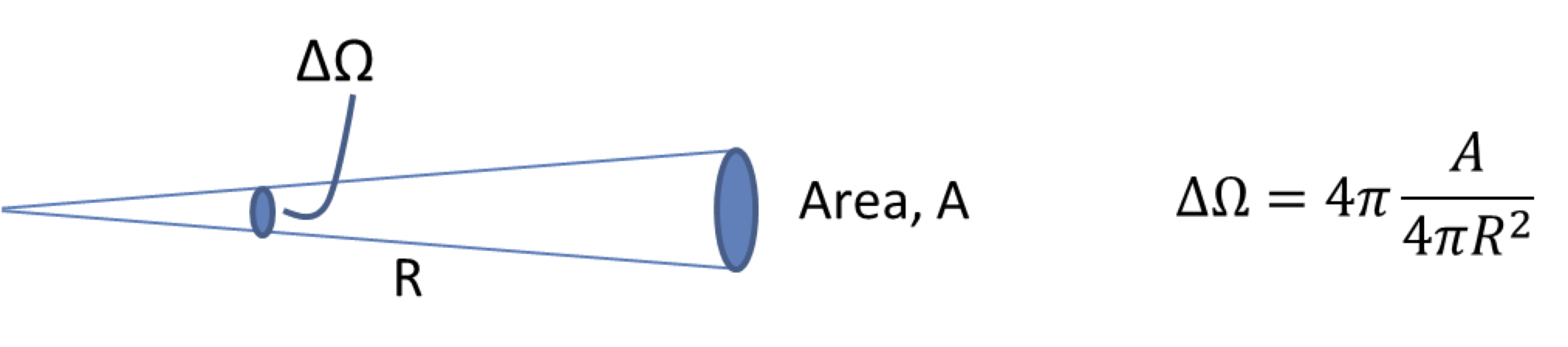## Section46.5Photometry

Radiometry gives us the objective measure of energy and power of light sources. However, human eye does not respond to all colors of light equally, and we perceive intensities differently than an objective instrument. For instance, experiments have shown that the eye is most sensitive to the yellow-green light than to the red and blue lights of the spectrum. Therefore, yellow-green light will appears brighter to our eyes than either the red or the blue light of the same intensity. To take the difference into account, a new set of physical measures of light is introduced for visible lights. these new metrics are called photometric quantities.

In the photometric quantities, power is weighted according to the human response by multiplying the radiometric quantity by a special function, called spectral luminance efficiency or the $V(\lambda)$ function, whose value is between 0 and 1. The $V$ function takes care of the response of human eye to various wavelengths. The $V$-function was arrived at experimentally in 1924, and is an average response of a population of people in a wide range of ages. The astronomical instruments use a $V$-filter for photometry.

The units as well as the names photometrics quantities differ from those in radiometry. For instance, power is simply called power in radiometry, but it is called the luminous flux in photometry, and while the unit of power in radiometry is $\text{Watt}\text{,}$ in photometry it is $\text{lumen}\text{.}$ A $\text{lumen}$ is defined in terms of a superfluous fundamental unit, called candela which is one of the seven independent quantities of the SI system of units, viz. meter, kilogram, second, ampere, Kelvin, mole and candela.

Candela is the SI unit of the photometric quantity called luminous intensity or luminosity that corresponds to the radiant intensity in radiometry. The following working definition was adopted for candela in 16th General Conference on Weights and Measures in 1979.

A monochromatic radiation source of frequency $540 \times 10^{12}\text{ Hz}$ is said to have a luminous intensity of one candela in a given direction if it emits $\frac{1}{683}\text{ watt}$ of power per steradian in that direction.

Since there are $\frac{4}{pi}$ steradians in the full solid angle around a source, the total power emitted by a one-candela source is $\frac{4}{683\pi}\text{ W}\text{,}$ which is equal to $1.0\text{ lumen}\text{,}$ if the source emits radiation isotropically in all directions.

\begin{equation} 1.0 \text{ lumen} = \frac{4}{683\pi}\text{ W}.\tag{46.5.1} \end{equation}

Table 46.5.1 shows the units of radiometry and photometry side by side. Various sources of light are often labelled with their luminance, which is the photometric term for the total power radiated per unit are per unit solid angle. Table 46.5.2 gives approximate luminance of several sources of light.

What will be the luminance of moon if observed from half the distance to the moon? Luminance of Moonlight on Earth $= 3000\text{ nits}\text{.}$

Hint

Luminosity varies as inverse square of distance from an isotropic source. Assume moon as an isotropic source.

$1.2 \times 10^4\text{ nits}\text{.}$

Solution

The luminosity will vary in space from the source (moon here) as the intensity varies. Let us assume moon to be an isotropic source of secondary light. Therefore, the luminosity at half the distance will be 4 times as much.

\begin{equation*} L_{x/2} = \left(\dfrac{x}{x/2} \right)^2 \times L_x = 4\times 3000 = 12,000\:\text{nit}. \end{equation*}

The luminance of a laptop monitor is $150\text{ nits}$ at the eye $25\text{ cm}$ from the screen. How much energy in Joules enters the eye in one hour if the cross-section of the pupil is assumed to be a circle of radius $5\text{ mm}\text{?}$

Hint

$1.55 \times 10^{-5}\text{ J}\text{.}$

Solution

The solid angle $\Delta\Omega$ subtended at the cross-section of the pupil by a point source 25 cm from the eye is given by $4\pi$ times the ratio of the area of the eye to the area of the spherical surface from the source.Figure 46.5.5. The solid angle and area element at the eye for Checkpoint 46.5.4.
\begin{equation*} \Delta\Omega = \dfrac{ \pi (0.5\:\text{cm})^2}{(25\:\text{cm})^2} = 2.5\times 10^{-4}\:\text{sr}, \end{equation*}

and the luminosity given in nits can be expressed in SI units to give

\begin{equation*} 150\:\text{nit} = \dfrac{150}{683}\:\dfrac{\text{W}}{\text{m}^2\text{sr}}. \end{equation*}

Therefore,

\begin{align*} \text{Energy} \amp = \dfrac{150}{683}\:\dfrac{\text{W}}{\text{m}^2\text{sr}}\times 2.5\times 10^{-4}\:\text{sr} \times \pi(0.005\:\text{m})^2 \\ \amp = 1.55\times 10^{-5}\:\text{J}. \end{align*}

The irradiance of the sunlight on the Earth is approximately $1.4\text{ kW/m}^2\text{.}$ Determine the illuminance.

Hint

Use definition of illuminance.

$9.6 \times 10^5\text{ lx}\text{.}$

Solution

Using the definition of illuminance we get

\begin{equation*} \text{Illuminance} = 1.5\times 10^3\:\frac{\text{W}}{\text{m}^2}\times \dfrac{683\:\text{lux}}{1\:\text{W/m}^2} = 9.56\times 10^5\:\text{lux}. \end{equation*}

The irradiance of the sunlight on the Earth is approximately $1.4\text{ kW/m}^2\text{.}$ The average distance between the Earth and the Sun is $1.5\times10^8\text{ km}\text{.}$ Determine the radiant intensity and luminous intensity of the Sun.

Hint

Use definitions of radiant intensity and luminous intensity.

$3.15 \times 10^{22}\text{ kW/sr}\text{,}$ $2.15 \times 10^{28}\text{ cd}\text{.}$